# Dear Family, your Operation Maths guide to Lines and Angles

## Dear Family, your Operation Maths guide to Lines and Angles

Category : Uncategorized

Dear Family, below is a brief guide to understanding the topic of lines and angles as well as some practical suggestions as to how you might support your children’s understanding at home. Also below, are a series of links to digital resources that will help both the children, and you, learn more about lines and angles. The digital resources are organised according to approximate class level:

#### Understanding Lines and Angles

Line and angles is a strand unit in the Primary Maths Curriculum for 2nd class up. For most people, when they think about angles, they also think about degrees, for example a 90° (ninety degree) angle. Yet in primary school, we don’t introduce degrees, as a way to describe, measure and construct angles, until 5th class. So what are they doing before that?

Initially, children are exploring angles as ‘turns’ i.e. recognising angles in terms of rotation: quarter-turns, half-turns and full turns. The children explore this themselves by turning to show the different turns, in both clockwise (turning right) and anticlockwise (turning left) directions.

In school, the children will also be shown other real-world examples of angles, including angles made by the hands of a clock, by the blades of an open scissors, by a door opening and closing, etc. We also look at angles (or corners/vertices) in 2-D shapes, for example in rectangles and triangles… even the names of these shapes pay homage to the angles that made them what they are today! (triangle = tri (or 3) angle; rectangle = rect (right/proper) angle)

In third class, the children will begin to use the term right angle, as a more mathematically correct way to describe the quarter turn (when movement is involved) or square corner (when there is no movement i.e. the angle is static) that they met in second class. The children will also learn to identify and name a variety of angles: angles less than a right angle (acute angles), angles more than a right angle (obtuse or reflex, more than 2 right angles/a straight angle) and angles equal to 2 right angles (straight angle). Then, in 5th and 6th class, they will begin to use degrees to identify, measure and construct various types of angles. This requires the use of a protractor, from a maths set, and it is not the most obvious or straightforward tool to use, so plenty of practice is required (see video links below in the Digital resources section for 5th and 6th class).

You can’t have an angle without having at least two connecting lines, therefore lines are an integral part of this area of maths. Like angles, not all lines are the same and the children learn to recognise and describe lines as horizontal, vertical, oblique, parallel or perpendicular.

#### Practical Suggestions for Supporting Children

• Ask your child to teach you about the angles and lines in your home. What different types of lines can be seen? What different types of angles can be seen? Can they name them?
• Line Hunt: ask your child to show you some lines that they can see at home. Ask them to run their finger along the lines so they get a sense of the line’s direction and position.
• Make a right angle finder: From a scrap piece of paper, tear out a large circular shape. Fold the shape in two, and then fold it in two again. The two straight edges/lines meet at a corner to make a right angle. Ask your child:
• ‘Have you heard any other name for this type of angle?’ (square corner, quarter turn)
• ‘Can you find any angles this size in this room? What are they called?’ (right angles)
• ‘Can you find any angles greater than/smaller than right angles in this room?’
• What’s in name? Write out your name in all capital letters. Name the different line types and angle types you can see. Are there any lines of symmetry in the letters? How many lines of symmetry and where? Click here to see some possible answers.
• You gotta hand it to ’em! Look closely at your hand… can you see angles in the lines made by your fingers? Estimate the degrees and then click here to see possible answers.
• Programming If your child does programming, or is interested in trying it out, they could use a free programme such as Scratch to draw various line and angle types.

#### Digital Resources for Second to Fourth ClassesTurns, Angles and Lines: A series of video lessons from White Rose Maths, including for Year 2,  Describing Turns 1, Describing Turns 2. For Year 3: Right Angles in ShapesCompare Angles, Horizontal & Vertical, Parallel & Perpendicular. For Year 4: Identify Angles and Compare and Order Angles.Lines & Angles: A series of video lessons from Matholia including What are Angles?Right Angles, Identifying Angles, Parallel Lines and Perpendicular LinesKhan Academy – Right Angles: Read through and then answer the practice questions. Afterwards, for something more challenging, look at the Fourth Grade Activities, which explore different types of lines and angles. You can also register for a free Khan Academy account to record your progress and explore other topics/grades.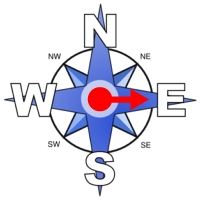Turns on a compass: Compare the start and end positions of the dial and decide how it turned.Turn the man: Explore how many times you need to turn the man to match the images.Turtle Diary: Learn about lines and angles and then take the quizzes! Parallel, perpendicular and intersecting lines; Types of Angles 1; Types of Angles 2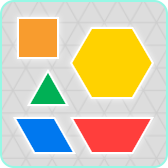Pattern Blocks: Explore the lines and angles that can be made with these interactive pattern blocks. Also includes a protractor feature for measuring the angles.Geoboard: Make lots of different angles and lines using this interactive geoboard, free from the Math Learning Centre.

Acute, Obtuse or Right angle: Answer the quiz questions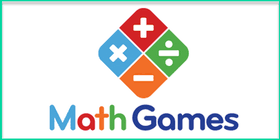Math Games: Identify the parallel, perpendicular and intersecting linesGeometry: a selection of games from ixl.com, including types of angles, obtuse, acute or straight, types of lines. You can do a number of free quizzes each day without having a subscription. (Please note that the class levels given do not always align accurately with the content of the Irish Primary Curriculum.)

#### Digital Resources for Fifth & Sixth ClassesAngles and Lines: A series of video lessons from White Rose Maths, including Measure with a Protractor, Introduce Angles, Angles in a TriangleKhan Academy – Measuring Angles: A unit of work exploring angles, including how to understand angles, how to measure angles and decomposing angles. Other relevant lessons include this one on the sum of angles in a triangle and this one on the sum of angles in a quadrilateral. You can also register for a free Khan Academy account to record your progress and explore other areas and/or try more difficult material.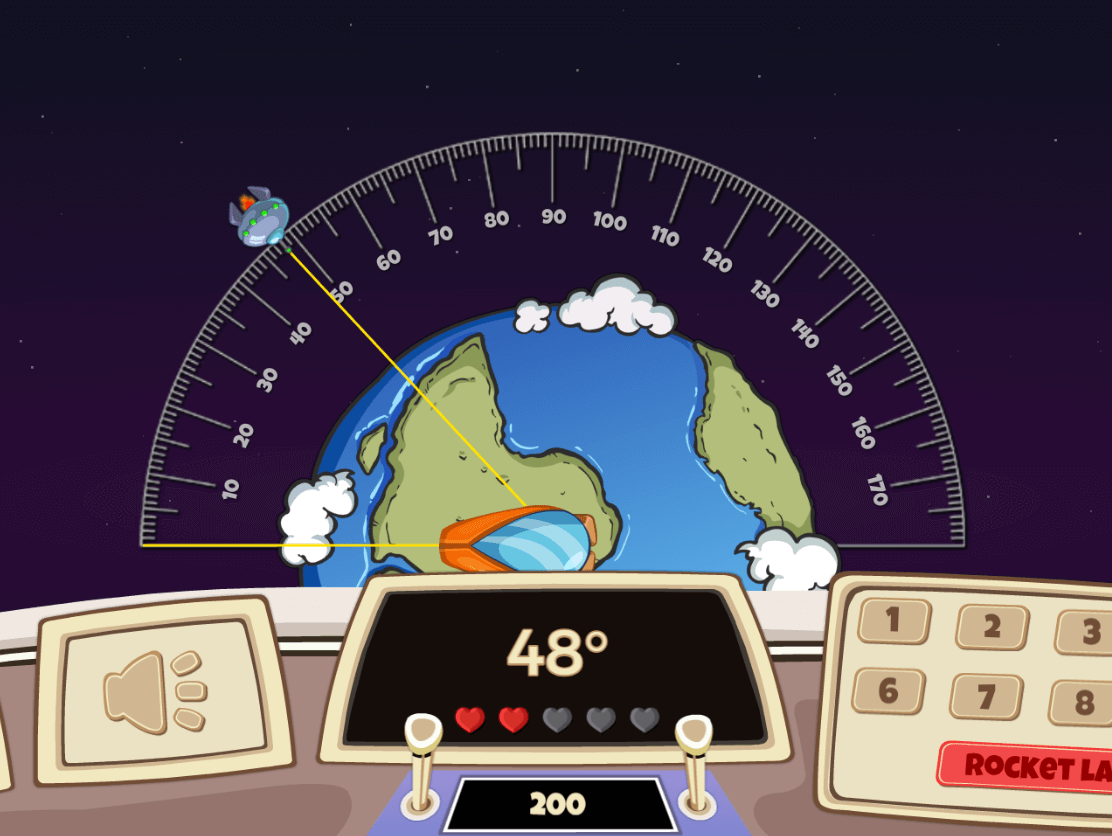Angle Alien Attack: Defend the Earth from an alien invasion using your knowledge of angles. Choose to read the angles from the protractor or estimate them without a protractor.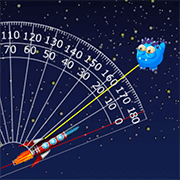Alien Angles: Create a specified angle to destroy the aliens. Challenging, but great for developing the ability to estimate angles. A similar game is Rocket Angles; this time you must estimate and input the measure of the given angle in degrees.Estimating Angles: In this game you must stop the angle size as near as possible to the target measure in degrees.Turtle Diary: Learn about lines and angles and then take the quizzes! Parallel, perpendicular and intersecting lines; Types of Angles 1; Types of Angles 2; Angles in Degrees; Estimating AnglesPattern Blocks: Explore the lines and angles that can be made with these interactive pattern blocks. Also includes a protractor feature for measuring the angles.Geoboard: Make lots of different angles and lines using this interactive geoboard, free from the Math Learning Centre.That Quiz – Angles: This quiz has lots of options, on the left hand side, that can be changed to suit the ability of the child. Ensure that the level is set to 1. Each time do the set 10 questions, if you get 10 or 9 correct go up a level, if not stay at that level. Start with only the “Measure” option on the left-hand side ticked, and when you bring the mouse across the screen, it changes into a transparent protractor. Other options included calculating the value of a missing angle in a triangle, (Triangle), and calculating the value of a missing angle in intersecting lines  (Line) or parallel lines (Parallel).Geometry: a selection of games from ixl.com, including classifying triangles and quadrilaterals. You can do a number of free quizzes each day without having a subscription. (Please note that the class levels given do not always align accurately with the content of the Irish Primary Curriculum.)Math Games: selection of interactive geometry quizzes, that includes lines and angles.